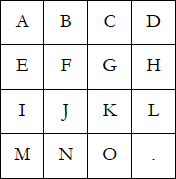### COCI 2006/2007, Contest #3

N-puzzle is a puzzle that goes by many names and has many variants. In this problem we will use the 15-puzzle. It consists of a 4-by-4 grid of sliding squares where one square is missing. The squares are labeled with uppercase letters 'A' through 'O', with the desired layout as follows:It can be useful (for example, when solving the puzzle using a computer) to define the "scatter" of a puzzle as the sum of distances between each square's current position and its position in the desired layout. The distance between two squares is their Manhattan distance (the absolute value of the sum of differences between the two rows and the two columns).

Write a program that calculates the scatter of the given puzzle.

### Input

Four lines of input contain four characters each, representing the state of the puzzle.

### Output

Output the scatter of the puzzle on a single line.

```ABCD
EFGH
IJKL
M.NO
```

```2
```

```.BCD
EAGH
IJFL
MNOK
```

### Output

```6
```

Point Value: 5
Time Limit: 1.00s
Memory Limit: 32M

Languages Allowed:
C++03, PAS, C, HASK, ASM, RUBY, PYTH2, JAVA, PHP, SCM, CAML, PERL, C#, C++11, PYTH3

• (8/3)
Hello,

My code, run locally, gets all of the right answers. (I peeked at the test data.) However, on your servers, it gets all WAs. All of my answers have been shifted up by 74. I am running in Python 2/3.

I got ACs by subtracting 74 from everything.

Regards,

bobhob314

• (2/0)